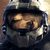+1
Спасибо

# Accumulation of double precision vector

dattorro 1 год назад обновлен Pavel Holoborodko 1 год назад

Quadruple precision floating-point accumulation of a double precision vector  p  is emulated numerically in Matlab:

```function t = orosumvec(p, recurs)
x = cumsum(p);
z = x - p;
u = (z - x) + p;
v = [0
x(1:end-1)] - z;
if ~recurs
t = sum([u
v
x(end)]);
else
t = orosumvec([u
v
x(end)], 0);
end
end```

This is verified by Advanpix Multiprecision Toolbox.

Matlab's built-in variable precision arithmetic, vpa() from Mathworks Symbolic Math Toolbox,

produces erroneous results in 2018One recursive call is necessary and sufficient.Спасибо

Thank you very much for the report!

Could you please provide full example, so that other people can easily copy and reproduce the situation?

If you want, we can add this example to the VPA vs. MCT comparison here:

Symbolic Math Toolbox (VPA) vs. Multiprecision Computing Toolbox